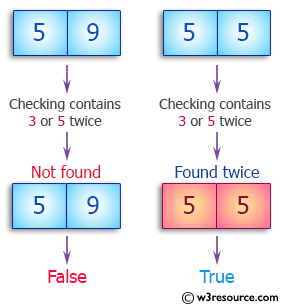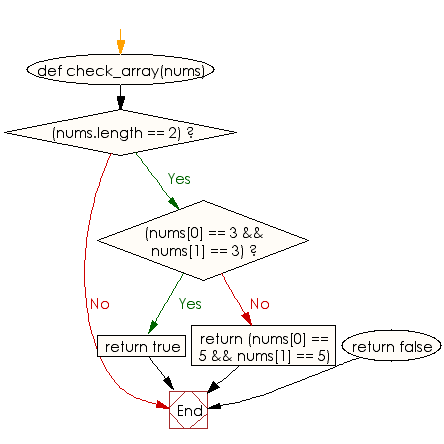﻿ Ruby Array exercises: Check whether a given array of integers contains 3 twice, or 5 twice - w3resource# Ruby Array Exercises: Check whether a given array of integers contains 3 twice, or 5 twice

## Ruby Array: Exercise-19 with Solution

Write a Ruby program to check whether a given array of integers contains 3 twice, or 5 twice. The array will be length 0, 1, or 2.Ruby Code:

``````def check_array(nums)
if(nums.length == 2)
if(nums == 3 && nums == 3)
return true
else
return (nums == 5 && nums == 5)
end
return false
end
end
print check_array([3, 3]),"\n"
print check_array([3, 6]),"\n"
print check_array([5, 9]),"\n"
print check_array([5, 5]),"\n"
print check_array([8, 9])
``````

Output:

```true
false
false
true
false
```

Flowchart:Ruby Code Editor: